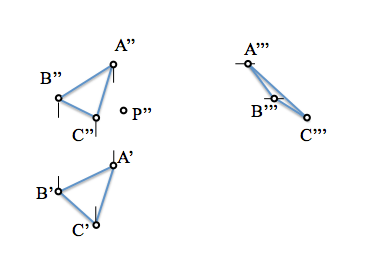# Diédrico System: Projection of the planeAfter seeing the System fundamentals Diédrico, with the projection of a point on two planes orthogonal projection, separating the land line system when we have two or more points, We have seen how obtain the projection of a line and determination of the third projection of a segment.

A plane is determined by three unaligned points, so adding a new point to a straight line projections can define it. In this case we will give at least two related dimensions on each plane of projection in order to become independent projections of these plans support of representation.We see that, again, the projection of the plane on two parallel planes is invariant in the case of cylindrical projection (This orthogonal if).

Just as we saw with the projections of the straight line, diedricas of a plane projections shall be sufficiently specific with the projection of the plane about two others that form a dihedral system, namely, they are orthogonal. Normal will give projections on a vertical plane and other horizontal, but it would be equally possible to give a vertical and a profile.From these two projections is very easy to determine the third on a new plane orthogonal to the former as, as in the determination of the third projection of the straight line, the dimensions shall be kept (z), departures (and) and deviations (x) with respect to the planes of projection.If the plane is determined by three points (or two straight lines that cut) We can find the projections in the three representations (Horizontal, Vertical and profile) new points or lines belonging to it.Can you get from a projection of a point belonging to a plane the other two projections on the remaining flat diedricos? But the point seems to be out of the plane don't be fooled, the plane is infinite.